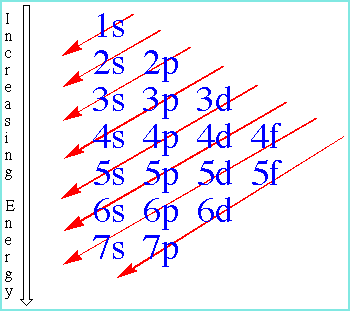Knowledge database: Basics: Electrons To understand why and how the atoms of different elements bond, it is necessary to know more about the atomic structure. If one looks at the periodic table of elements (PTE), one can see that each atom has its (atomic) number. This number tells how many protons an atom has in its nucleus, and how many electrons there are in the electron cloud. Hydrogen has one proton and one electron, which is also located in his last (and only) orbital - an energy level containing electrons. The next atom in line, helium, has 2 electrons, which are still in a single energy level (orbital). Orbitals can, for simplicity, be imagined as rings around the nucles in which the electrons circulate. In these rings 2 electrons can be placed (s orbital). Therefore, with the next element in the row, lithium, the first ring is filled, and the second ring (also an s orbital), contains only 1 electron. To avoid describing this element by element, the scheme by which electrons are placed in orbitals can be seen below.Electron placement scheme. As one can see, there are s, p, d and f orbitals. The s orbitals hold 2 electrons, p orbitals hold 6, d orbitals 10, and f orbitals holds 14 electrons. When one fills the 1s orbital, one goes on to the 2s orbital. After the 2s orbital is full, next comes the 2p orbitals (which unlike the s orbitals, hold 6 electrons). It is also necessary to describe exactly how the electrons are filled into the orbitals (picture below).Electron positioning method. Each square has "room" for 2 electrons. Since the s orbital receives only 2 electrons, only one box is shown. P orbitals are represented with 3 squares because they can recieve 6 electrons, etc. It is also very important to mention that the electrons are first filled by introducing 1 electron per square, and when all squares have one electron, only then are electrons introduced to squares where 1 electron is already located. For an example, one should take a look at the d orbitals in the picture above. There are 6 electrons which have filled the squares of d orbitals. The first 5 are deployed so that one is placed in each square, and then the sixth electron is placed in the first box along with the electron which has previously been placed there. By following these rules one can fill the orbitals of any atom/element. This is important because by knowing the number of free, unmatched electrons (those that stand alone in a square of some of the last orbitals) one can determine the oxidation numbers of an atom. More on this in the next section.